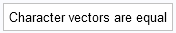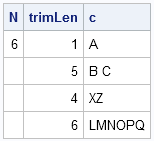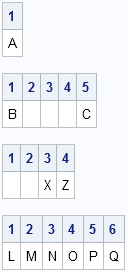SAS programmers are probably familiar with how SAS stores a character variable in a data set, but how is a character vector stored in the SAS/IML language?

Recall that a character variable is stored by using a fixed-width storage structure. In the SAS DATA step, the maximum number of characters that can be stored in a variable is determined when the variable is initialized, or you can use the LENGTH statement to specify the maximum number of characters. For example, the following statement specifies that the NAME variable can store up to 10 characters:

```data A; length name \$ 10; /* declare that a variable stores 10 characters */ ...```

The values in a character variable are left aligned. That is, values that have fewer than 10 characters are padded on the right with blanks (space characters).

### SAS/IML character vectors

The same rules apply to character vectors in the SAS/IML language. A vector has a "length" that determines the maximum number of characters that can be stored in any element. (In this article, "length" means the maximum number of characters, not the number of elements in a vector.) Elements with fewer characters are blank-padded on the right. Consequently, the following two character vectors are equivalent. :

```proc iml; c = {"A", "B C", " XZ", "LMNOPQ"}; /* length set at initialization */ c2 = {"A ", "B C ", " XZ ", "LMNOPQ"}; /* all strings have length 6 */ if c=c2 then print "Character vectors are equal"; else print "Character vectors are not equal";```You can determine the maximum number of characters that can be stored in each element by using the NLENG function in SAS/IML. You can also discover the number of characters in each element of a vector (omitting any blank padding) by using the LENGTH function, as follows:

```N = nleng(c); trimLen = length(c); print N trimLen c;```In this example, each element of the vector c can hold up to six characters. If you write the c variable to a SAS data set, the corresponding variable will have length 6. However, if you trim off the blanks at the end of the strings, most elements have fewer than six characters. Notice that the LENGTH function counts blanks at the beginning and the middle of a string but not at the end, so that the string "  XZ" counts as four characters.

### Where are the blanks?

Notice that the ODS HTML destination is not ideal for visualizing blanks in strings. In HTML, multiple blank characters are compressed into a single blank when the string is rendered, so only one space appears on the displayed output. If you need to view the spaces in the strings, use the ODS LISTING destination, which uses a fixed-width font that preserves spaces. Alternatively, the following SAS/IML function prints each character (not including trailing blanks):

```/* convert a string to a row vector of single characters (uses SAS/IML 12.1) */ start Str2Vec(s); return (substr(s, 1:length(s), 1)); /* row vector of characters */ finish;   /* print characters of all strings in a vector */ start PrintChars(v); L = length(v); /* characters per name, not counting trailing blanks */ do i = 1 to ncol(L)*nrow(L); c = char(1:L[i], 2); print (Str2Vec(v[i]))[colname=c]; /* print individual letters */ end; finish;   run PrintChars(c);```I think the Str2Vec function is very cool. It uses a feature of the SUBSTR function in SAS/IML 12.1 to convert a string into a vector of characters. The PrintChars function simply calls the Str2Vec function for each element of a character matrix and prints the characters with a column header. This makes it easy to see each character's position in a string.

This article provides a short overview of how strings are stored inside SAS/IML character vectors. For more details about SAS/IML character vectors and how you can manipulate strings, see Chapter 2 of Statistical Programming with SAS/IML Software.

ShareDistinguished Researcher in Computational Statistics

Rick Wicklin, PhD, is a distinguished researcher in computational statistics at SAS and is a principal developer of PROC IML and SAS/IML Studio. His areas of expertise include computational statistics, simulation, statistical graphics, and modern methods in statistical data analysis. Rick is author of the books Statistical Programming with SAS/IML Software and Simulating Data with SAS.

1.•